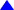Econ 2630                           Study Guides: Ch 22 thru 25                         Dr. Usip

Study Guide for Ch 22
You should know the following:

1.    Explain the usefulness of the short-run macro model.

2.    List the four components of aggregate expenditure included in the simple macro model of this chapter.

3.    List the determinants of consumption spending and describe their effects on consumption spending.

4.    Describe a consumption function in terms of autonomous consumption and the marginal propensity to consume.

5.    Show how changes in taxes and in autonomous consumption changes affect the consumption-income line.

6.    Explain why inventory investment is not included in aggregate expenditure.

7.    Define government spending.

8.    Define net exports.

9.    Explain why investment, government spending, and net exports are assumed to be determined by forces outside the short-run macro model.

10.    Use a 45° translator line, together with the aggregate expenditure line, to show inventory changes and to find the short-run equilibrium output level.

11.    Explain why short-run equilibrium output is not necessarily full-employment output.

12.    Use the multiplier to show how a spending shock affects equilibrium output in an economy.

13.    Give examples of automatic stabilizers and explain how they reduce the impact of spending shocks.

14.    Describe how spending and saving play different roles in the classical model and in the short-run macro model.

15.    Use the short-run macro model of the chapter to explain the causes of the 1990–91 recession.

Study Guide for Ch. 23
You should know the following:

1.    Define liquidity.

2.    Explain why credit cards, gold bars, and stocks and bonds are not money.

3.    List and define the components of M1 and M2.

4.    Describe a bank’s balance sheet.

5.    Describe the structure and functions of the Federal Reserve System.

6.    List the tools that the Fed can use to change the money supply.

7.    Explain how the Fed uses open market operations to change the nation’s money supply.

8.    Show how the demand deposit multiplier is used to calculate the total change in demand deposits resulting from an initial change in reserves.

9.    Explain how the size of the demand deposit multiplier is affected by the public’s desire to hold cash and banks’ desires to hold excess reserves.

10.    Describe how the Fed affects the money supply when it changes the required reserve ratio or the discount rate, and explain why the Fed seldom uses these two tools.

11.    Explain why it is important to prevent bank panics, and why there have been relatively few bank failures since 1933.

Study Guide for Ch. 24
You should know the following:

1.    Explain how individuals’ wealth constraints limit their demand for money.

2.    Describe the tradeoff between holding money and bonds.

3.    List the key variables that affect one’s demand for money and explain its effects.

4.    Draw a money demand curve and explain what causes movements along it and what causes shifts to a new money demand curve.

5.    Draw a money supply curve and explain why it is vertical.

6.    State the relationship between bond prices and the interest rate, and use this relationship to explain how the money market reaches equilibrium.

7.    Describe how the Fed uses open market purchases to control the interest rate, and explain how this affects equilibrium GDP.

8.    Use the crowding-out effect to explain why interest rate changes diminish the effectiveness of fiscal policy and other spending shocks.

9.    Describe why the loanable funds market model, which determines the interest rate in the long-run classical view, does not determine the interest rate in the short-run macro view.

10.    Explain how a change in expectations about future interest rates shifts the money demand curve.

11.    Explain how the Fed saved the economy from a more severe and longer lasting recession in 2001.

Study Guide for Ch. 25
You should know the following:

1.      Use an aggregate demand (AD) curve to illustrate how price level changes affect equilibrium GDP.

2.    List the variables that cause the AD curve to shift and explain their effects.

3.    Use an aggregate supply (AS) curve to illustrate how a change in output affects the price level in the short run, through its impact on unit costs.

4.    Explain why, when the AS curve is derived, the nominal wage rate is assumed to be fixed.

5.    List the variables that cause the AS curve to shift, and explain their effects.

6.    Use an AD–AS diagram to show how an economy arrives at a point of short-run equilibrium.

7.    Explain why the long-run AS curve is vertical.

8.    Describe the economy’s self-correcting mechanism.

9.    Explain how demand shocks affect the economy in the short run and in the long run.

10.    Explain how supply shocks affect the economy in the short run and in the long run.

11.    Define stagflation.

Remember: Final Exam is on Thursday, Dec. 14, 10:30am - 12:30pmTop or Return to Course Information or Home Page or Send me Comments via E-mail